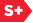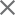### Proceedings Paper

Affine scaling transformation algorithms for harmonic retrieval in a compressive sampling framework
Author(s): Sergio D. Cabrera; Jose Gerardo Rosiles; Alejandro E. Brito
Format Member Price Non-Member Price
PDF \$17.00 \$21.00

Paper Abstract

In this paper we investigate the use of the Affine Scaling Transformation (AST) family of algorithms in solving the sparse signal recovery problem of harmonic retrieval for the DFT-grid frequencies case. We present the problem in the more general Compressive Sampling/Sensing (CS) framework where any set of incomplete, linearly independent measurements can be used to recover or approximate a sparse signal. The compressive sampling problem has been approached mostly as a problem of l1 norm minimization, which can be solved via an associated linear programming problem. More recently, attention has shifted to the random linear projection measurements case. For the harmonic retrieval problem, we focus on linear measurements in the form of: consecutively located time samples, randomly located time samples, and (Gaussian) random linear projections. We use the AST family of algorithms which is applicable to the more general problem of minimization of the lp p-norm-like diversity measure that includes the numerosity (p=0), and the l1 norm (p=1). Of particular interest in this paper is to experimentally find a relationship between the minimum number M of measurements needed for perfect recovery and the number of components K of the sparse signal, which is N samples long. Of further interest is the number of AST iterations required to converge to its solution for various values of the parameter p. In addition, we quantify the reconstruction error to assess the closeness of the AST solution to the original signal. Results show that the AST for p=1 requires 3-5 times more iterations to converge to its solution than AST for p=0. The minimum number of data measurements needed for perfect recovery is approximately the same on the average for all values of p, however, there is an increasing spread as p is reduced from p=1 to p=0. Finally, we briefly contrast the AST results with those obtained using another l1 minimization algorithm solver.

Paper Details

Date Published: 20 September 2007
PDF: 12 pages
Proc. SPIE 6701, Wavelets XII, 67012D (20 September 2007); doi: 10.1117/12.735139
Show Author Affiliations
Sergio D. Cabrera, The Univ. of Texas at El Paso (United States)
Jose Gerardo Rosiles, The Univ. of Texas at El Paso (United States)
Alejandro E. Brito, Xerox Corp. (United States)

Published in SPIE Proceedings Vol. 6701:
Wavelets XII
Dimitri Van De Ville; Vivek K. Goyal; Manos Papadakis, Editor(s)